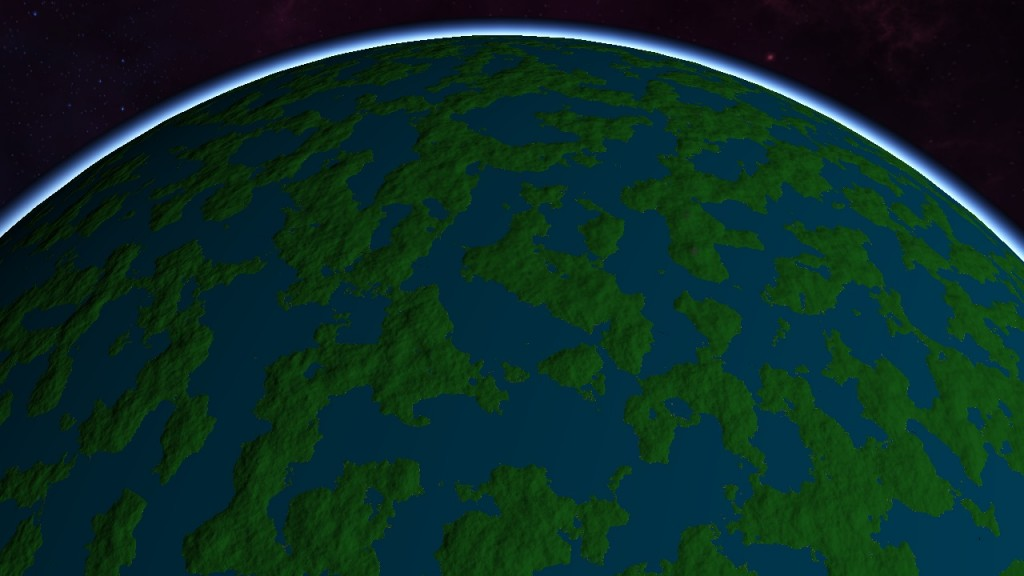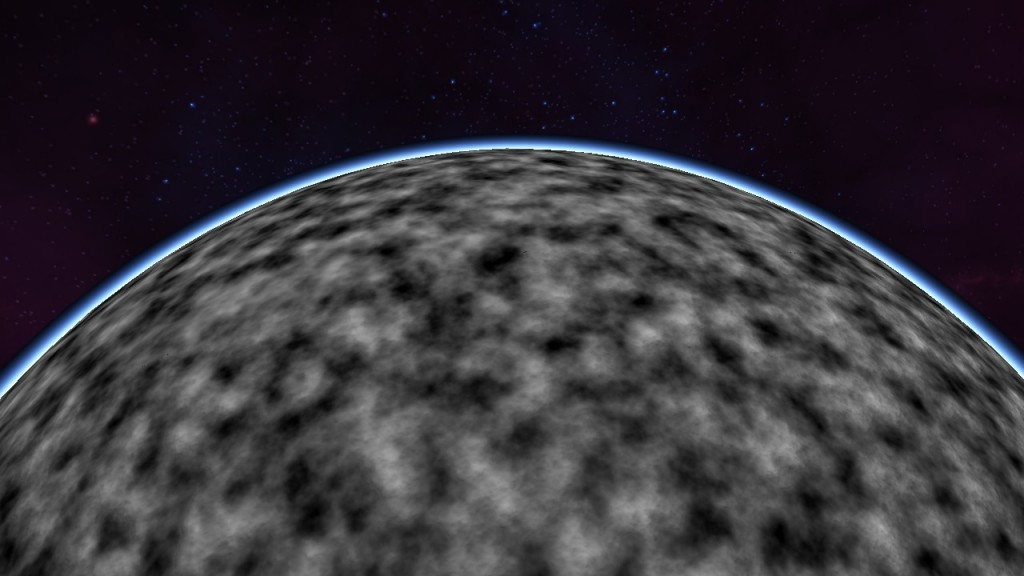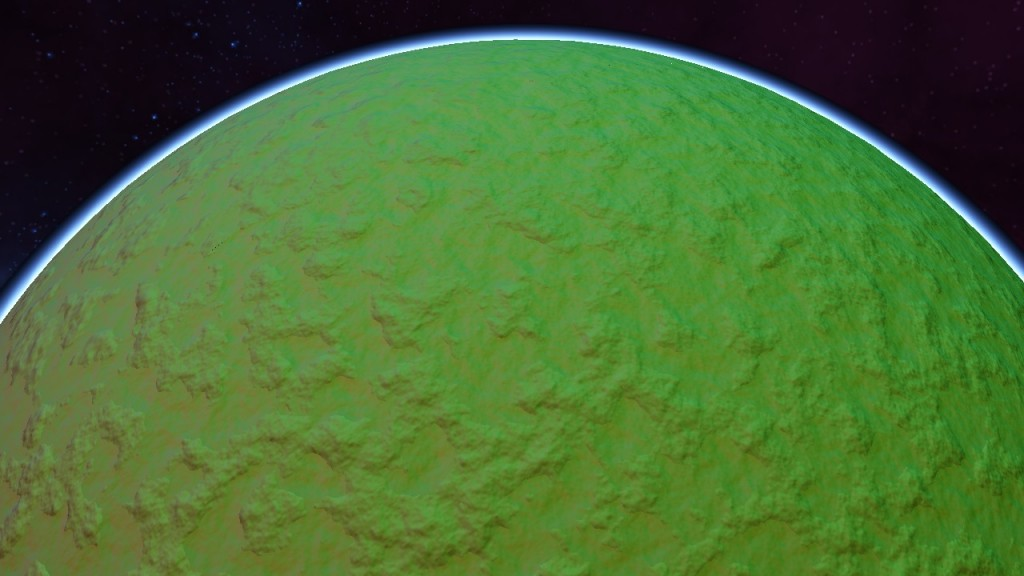# Compute TexturingOriginally I was computing the height of terrain per frame which was pretty wasteful since if I wanted more complex noise functions I would not be able to maintain a runnable frame rate. I moved the computation of terrain height to a compute shader which stores the height in a texture. I was also able to compute and store normals in the rgb space of the texture.

In order to create a unique texture for each node I create a cache that holds all of the textures that a node may use in a map using an unsigned long long to give each texture a unique ID.

As the tree is traversed downwards, the nextId is passed to the next recursive call and used as the currentId. The QUADRANT_ID is an integer from 0 to 3 which identifies the quadrant that is being split. The ID is added onto using bit shifting using 3 bits to describe each LOD level where the first two bits are used to describe the quadrant and the last bit is used to give each node a unique ID even if it has child nodes.

At some point I will probably switch to just holding a tree structure and splitting/joining nodes every frame in order to remove the need for unique IDs since at the moment the maximum number of levels of detail that can be stored is 21 because long long‘s only have 64 bits to work with.The terrain height map is generated using the same fractional Brownian motion function that I described in my earlier post. The calculated height is stored in a 2D shared float array in the compute shader which is the size of each group. Prior to the normal calculation, the height value is stored in the shared float array named workGroupHeight.

```workGroupHeight[gl_LocalInvocationID.x][gl_LocalInvocationID.y] = heightValue;
```The normal calculation in this step is done in a second pass on the compute shader that generates out the height map. Once the height values are calculated and saved to a temporary 2D array of floats I call the GLSL function barrier(). Since GPUs are highly parallel, the processing for each pixel of the height map is separated into its own thread so it is possible for some threads to finish sooner than others. This is problematic since the normal computation step requires knowledge of the neighboring pixels’ height and if other threads query the neighboring pixel values before they have been computed in other threads then you can get sections of pixels that do not have correct normals. The call of the function barrier() makes all of the threads wait once they reach the barrier until every other thread has finished, then the program continues in parallel. I was not calling the correct barrier function early on, and instead used one of the memory barriers which resulted in a bar code pattern of normals that faced toward the center of the planet and normals that were correctly computed.

I calculate the normals by using the cross product of the point that the shader is currently working on and the vectors from this point to its two neighbors at (x+1, y) and (x, y+1) on the texture. Once the normals have been calculated I save the final normal and height values to an rgba32f texture using the GLSL function imageStore().

The normals that I generate in this step are stored in object space since this texture will only be used on this planet in this orientation. This has the advantage that I don’t need to work with tangent space and can just directly read the normal from the texture’s rgb, move the value stored in the texture to -1.0 – 1.0 from the 0.0-1.0 value stored in the texture, and use that resulting vector.